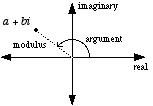index: click on a letter A B C D E F G H I J K L M N O P Q R S T U V W X Y Z A to Z index index: subject areas numbers & symbols sets, logic, proofs geometry algebra trigonometry advanced algebra & pre-calculus calculus advanced topics probability & statistics real world applications multimedia entrieswww.mathwords.com about mathwords website feedback

 Polar Form of a Complex Number The polar coordinates of a complex number on the complex plane. The polar form of a complex number is written in any of the following forms: rcos θ + irsin θ, r(cos θ + isin θ), or rcis θ. In any of these forms r is called the modulus or absolute value. θ is called the argument.See also